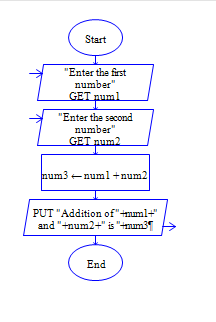# C Program to Addition of two numbers

Get two integer numbers, add both the integers and display the sum.

Sample Input 1:

5 6

Sample Output 1:

11

Sample Input 2:

65 4

Sample Output 2:

69

#### Flow Chart Design#### Program or Solution

``` #include<stdio.h> int main() { int a,b,c; scanf("%d %d",&a,&b); c=a+b; printf("%d",c); return 0; } ```

#### Program Explanation

Get two integers a and b (using scanf statement) Add a and b, then store the sum in c (c=a+b) print the value of c (using printf statement)